×#### Thank you for registering.

One of our academic counsellors will contact you within 1 working day.

Click to Chat

1800-1023-196

+91-120-4616500

CART 0

• 0

MY CART (5)

Use Coupon: CART20 and get 20% off on all online Study Material

ITEM
DETAILS
MRP
DISCOUNT
FINAL PRICE
Total Price: Rs.

There are no items in this cart.
Continue Shopping• Complete JEE Main/Advanced Course and Test Series
• OFFERED PRICE: Rs. 15,900
• View Details

```Chapter 10: Congruent Triangles Exercise – 10.1

Question: 1

In Fig. (10).22, the sides BA and CA have been produced such that: BA = AD and CA = AE. Prove that segment DE ∥ BC.Solution:

Given that, the sides BA and CA have been produced such that BA = AD and CA = AE and given to prove DE ∥ BC Consider triangle BAC and DAE,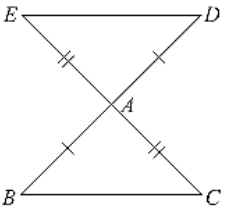We have

BA = AD and CA= AE                 [given in the data]

And also ∠BAC = ∠DAE             [vertically opposite angles]

So, by SAS congruence criterion, we have

∠BAC ≃ ∠DAE

BC = DE and ∠DEA = ∠BCA, ∠EDA = ∠CBA

[Corresponding parts of congruent triangles are equal]

Now, DE and BC are two lines intersected by a transversal DB such that ∠DEA = ∠BCA i.e..  alternate angles are equal Therefore, DE, BC ∥ BC.

Question: 2

In a PQR, if PQ = QR and L, M and N are the mid-points of the sides PQ, QR and RP respectively. Prove that LN = MN.

Solution:

Given that,

In PQR, PQ = QR and L, M, N are midpoints of the sides PQ, QP and RP respectively and given to prove that LN = MN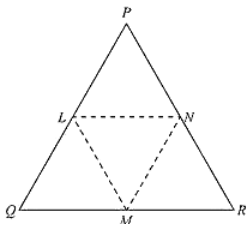Here we can observe that PQR is an isosceles triangle

PQ = QR and ∠ QPR = ∠ QRP  .... (i)

And also, L and M are midpoints of PQ and QR respectively

PL = LQ = QM = MR = PQ/2 = QR/2

And also, PQ = QR

Now, consider Δ LPN and Δ MRN, LP = MR              [From - (2)]

∠LPN = ∠MRN ... [From - (1)]

∠QPR and ∠LPN and ∠QRP and ∠MRN are same.

PN = NR                                                             [N is midpoint of PR]

So, by SAS congruence criterion, we have ΔLPN = ΔMRN

LN = MN [Corresponding parts of congruent triangles are equal]

Question: 3

In fig. (10).23, PQRS is a square and SRT is an equilateral triangle. Prove that (i) PT = QT (ii) ∠TQR = 15°Solution:

Given that PQRS is a square and SRT is an equilateral triangle. And given to prove that

(i) PT = QT and (ii) ∠TQR = 15°Now, PQRS is a square

PQ = QR = RS = SP ... (i)

And ∠SPQ = ∠PQR = ∠QRS = ∠RSP = 90° = right angle

And also, SRT is an equilateral triangle.

SR = RT = TS ... (ii)

And ∠TSR = ∠SRT = ∠RTS = 60°

From (i) and (ii)

PQ = QR = SP = SR = RT = TS  .... (iii)

And also,

∠TSP =  ∠TSR + ∠RSP = 60° + 90° + 150°

∠TRQ = ∠TRS + ∠SRQ = 60° + 90° + 150°

⟹ ∠TSR = ∠TRQ = 150°   ...  (iv)

SP = RQ           [From (iii)]

So, by SAS congruence criterion we have

ΔTSP = ΔTRQ

PT = QT   [Corresponding parts of congruent triangles are equal] Consider ΔTQR.

QR = TR        [From (iii)]

ΔTQR is a isosceles triangle.

∠QTR = ∠TQR [angles opposite to equal sides]

Now,

Sum of angles in a triangle is equal to 180∘

⟹ ∠QTR + ∠TQR + ∠TRQ = 180°

⟹ 2∠ TQR + 150° = 180°     [From (iv)]

⟹ 2∠TQR = 180° - 150°

⟹ 2∠ TQR = 30°                         ∠TQR = 15°] ...

Hence proved

Question: 4

Prove that the medians of an equilateral triangle are equal.

Solution:

Given,

To prove the medians of an equilateral triangle are equal.

Median: The line Joining the vertex and midpoint of opposite side.  Now, consider an equilateral triangle ABC.

Let D, E, F are midpoints of BC, CA and AB.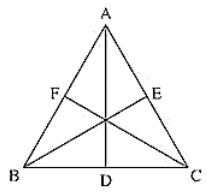Then, AD, BE and CF are medians of ABC.

Now,

D Is midpoint of BC ⟹ BD = DC = BC/2

Similarly, CE = EA = AC/2

AF = FB = AB/2

Since ΔABC is an equilateral triangle

⟹ AB = BC = CA  ... (i)

⟹ BD = DC = CE = EA = AF = FB = BC/2 = AC/2 = AB/2 .... (ii)

And also, ∠ABC = ∠BCA = ∠CAB = 60° ... (iii)

Now, consider ΔABD and ΔBCE AB = BC  [From (i)]

BD = CE  [From (ii)]

Now, in ΔTSR and ΔTRQ

TS = TR    [From (iii)]

∠ABD = ∠BCE            [From (iii)] [∠ABD and ∠ABC and ∠BCE and ∠BCA are same]

So, from SAS congruence criterion, we have

ΔABD = ΔBCE

[Corresponding parts of congruent triangles are equal]

Now, consider ΔBCE and ΔCAF, BC = CA                         [From (i)]

∠BCE = ∠CAF             [From (ii)]

[∠BCE and ∠BCA and  ∠CAF and ∠CAB are same]

CE = AF                [From (ii)]

So, from SAS congruence criterion, we have

ΔBCE = ΔCAF

BE = CF (v)

[Corresponding parts of congruent triangles are equal]

From (iv) and (v), we have

Median AD = Median BE = Median CF

The medians of an equilateral triangle are equal.

Hence proved

Question: 5

In a ΔABC, if ∠A = 120° and AB = AC. Find ∠B and ∠C.

Solution:

Consider a ΔABC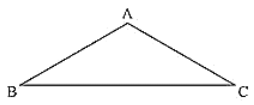Given Mat ∠A = 120° and AB = AC and given to find ∠B and ∠C.

We can observe that ΔABC is an isosceles triangle since AB = AC

∠B = ∠C (i)

[Angles opposite to equal sides are equal]

We know that sum of angles in a triangle is equal to 180°

⟹ ∠A + ∠B + ∠C = 180°                                    [From (i)]

⟹ ∠A + ∠B + ∠B = 180°

⟹ 120° + 2∠B = 180°

⟹ 2∠B = 180° - 120°

⟹ ∠B = ∠C = 30°

Question: 6

In a ΔABC, if AB = AC and ∠B = 70°. Find ∠A.

Solution:

Consider a ΔABC, if AB = AC and ∠B = 70°

Since, AB = AC ΔABC is an isosceles triangle

∠B = ∠C [Angles opposite to equal sides are equal]

∠B = ∠C = 70∘

And also,

Sum of angles in a triangle = 180°

∠A + ∠B + ∠C = 180°

∠A + 70° + 70° = 180°

∠A = 180° - 140°

∠A = 40°

Question: 7

The vertical angle of an isosceles triangle is (10)0°. Find its base angles.

Solution:

Consider an isosceles ΔABC such that AB = AC

Given that vertical angle A is (10)0°

To find the base angles

Since ΔABC is isosceles

∠B = ∠C [Angles opposite to equal sides are equal]

And also,

Sum of interior angles of a triangle = 180°

∠A + ∠B + ∠C = 180°

(10)0° + ∠B ∠B = 180°

2∠B = 180∘ - (10)0°

∠B = 40°

∠B = ∠C = 40°

Question: 8

In a ∆ABC = AC and ∠ACD = (10)5°. Find ∠BAC.

Solution:

We have,

AB = AC and ∠ACD = (10)5°

Since, ∠BCD = 180° = Straight angle

∠BCA + ∠ ACD = 180°

∠BCA + (10)5° = 180°

∠BCA = l80° - (10)5°

∠BCA = 75°

And also,

ΔABC is an isosceles triangle      [AB = AC]

∠ABC = ∠ ACB [Angles opposite to equal sides are equal]

From (i), we have

∠ACB = 75°

∠ABC = ∠ACB = 75°

And also,

Sum of Interior angles of a triangle = 180°

∠ABC = ∠BCA + ∠CAB = 180°

75° + 75° + ∠CAB =180°

150° + ∠BAC = 180°

∠BAC = 180° - 150° = 30°

∠BAC = 30°

Question: 9

Find the measure of each exterior angle of an equilateral triangle.

Solution:

Given to find the measure of each exterior angle of an equilateral triangle consider an equilateral triangle ABC.

We know that for an equilateral triangle

AB = BC = CA and ∠ABC = ∠BCA = CAB =180°/3 = 60° .... (i)

Now,

Extend side BC to D, CA to E and AB to F.

Here BCD is a straight line segment

BCD = Straight angle =180°

∠BCA + ∠ACD = 180° [From (i)]

60° + ∠ACD = 180°

∠ACD = 120°

Similarly, we can find ∠FAB and ∠FBC also as 120° because ABC is an equilateral triangle

∠ACD = ∠EAB - ∠FBC = 120°

Hence, the median of each exterior angle of an equilateral triangle is 120°

Question: 10

If the base of an isosceles triangle is produced on both sides, prove that the exterior angles so formed are equal to each other.

Solution:

ED is a straight line segment and B and C an points on it.

∠EBC = ∠BCD = straight angle = 180°

∠EBA + ∠ABC = ∠ACB + ∠ACD

∠EBA = ∠ACD + ∠ACB - ∠ABC

∠EBA = ∠ACD [From (i) ABC = ACD]

∠ABE = ∠ACD

Hence proved

Question: 11

In Fig. (10).2(5) AB = AC and DB = DC, find the ratio ∠ABD: ∠ACD.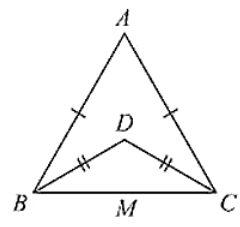Solution:

Consider the figureGiven,

AB = AC, DB = DC and given to find the ratio

∠ABD = ∠ACD

Now, ΔABC and ΔDBC are isosceles triangles since AB = AC and DB = DC respectively

∠ABC = ∠ACB and ∠DBC = ∠DCB [Angles opposite to equal sides are equal]

Now consider,

∠ABD : ∠ACD

(∠ABC - ∠DBC): (∠ACB - ∠DCB)

(∠ABC - ∠DBC): (∠ABC - ∠DBC) [∠ABC = ∠ACB and ∠DBC = ∠DCB]

1: 1

ABD: ACD = 1: 1

Question: 12

Determine the measure of each of the equal angles of a right-angled isosceles triangle. OR

ABC is a right-angled triangle in which ∠A = 90° and AB = AC. Find ∠B and ∠C.

Solution:

ABC is a right angled triangle

Consider on a right - angled isosceles triangle ABC such that

∠A = 90° and AB = AC Since,

AB = AC ⟹ ∠C = ∠B  .... (i)

[Angles opposite to equal sides are equal]

Now, Sum of angles in a triangle = 180°

∠A + ∠B + ∠C =180°

⟹ 90° + ∠ B+ ∠ B = 180°

⟹ 2∠B = 90°

⟹ ∠B = 45°

∠B = 45°, ∠C = 45°

Hence, the measure of each of the equal angles of a right-angled Isosceles triangle Is 45°

Question: 13

AB is a line segment. P and Q are points on opposite sides of AB such that each of them is equidistant from the points A and B (See Fig. (10).26). Show that the line PQ is perpendicular bisector of AB.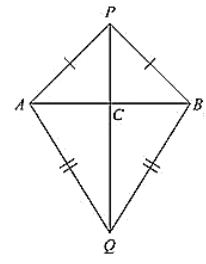Solution:

Consider the figure.We have

AB is a line segment and P, Q are points on opposite sides of AB such that

AP = BP    ... (i)

AQ = BQ     ... (ii)

We have to prove that PQ is perpendicular bisector of AB.

Now consider ΔPAQ and ΔPBQ,

We have

AP = BP                    [From (i)]

AQ = BQ                   [From (ii)]

And PQ - PQ             [Common site]

Δ PAQ ≃ Δ PBQ  ... (iii)  [From SAS congruence]

Now, we can observe that APB and ABQ are isosceles triangles. [From (i) and (ii)]

∠ PAB = ∠ ABQ and ∠ QAB = ∠ QBA

Now consider Δ PAC and Δ PBC

C is the point of intersection of AB and PQ

PA = PB                       [From (i)]

∠ APC = ∠ BPC           [From (ii)]

PC = PC                        [common side]

So, from SAS congruency of triangle ΔPAC ≅ ΔPBC

AC = CB and ∠PCA = ∠PBC ... (iv)   [Corresponding parts of congruent triangles are equal]

And also, ACB is line segment

∠ACP + ∠ BCP = 180°

∠ACP = ∠PCB

∠ACP = ∠PCB = 90°<

We have AC = CB ⟹ C is the midpoint of AB

From (iv) and (v)

We can conclude that PC is the perpendicular bisector of AB

Since C is a point on the line PQ, we can say that PQ is the perpendicular bisector of AB.
```### Course Features

• 728 Video Lectures
• Revision Notes
• Previous Year Papers
• Mind Map
• Study Planner
• NCERT Solutions
• Discussion Forum
• Test paper with Video Solution